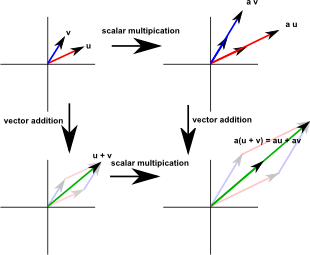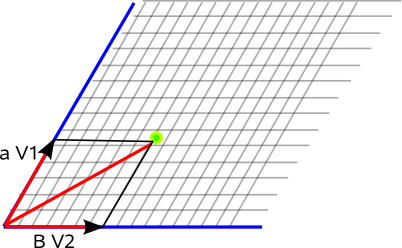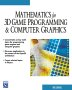# Maths - Vectors

Like many mathematical concepts, vectors can be understood and investigated in different ways.

There are at least two ways to look at vectors:

• Algebraic - Treats a vector as set of scalar values as a single entity with addition, subtraction and scalar multiplication which operate on the whole vector.
• Geometric - A vector represents a quantity with both magnitude and direction.

We can abstract away the differences in these approaches and just look at what is always true of vectors, when we do that we get a set of axioms usually in the form of equations. An example of an axiom for vectors is the 'distributivity law':

 c(v1 + v2) = c v 1 + c v2 where v 1 and v2 are vectors and c is a scalar.

This axiom is important because it describes the linear property of vectors

### Geometric Properties

A vector is a quantity with both magnitude and direction, there are two operations defined on vectors and these both have a very direct geometric interpretation. We draw a vector as a line with an arrow, for now I will call the end without the arrow the 'start' of the vector and the end with the arrow the 'end' of the vector.

• Vector addition: to add two vectors we take the start of the second vector and move it to the end of the first vector. The addition of these two vectors is the vector from the start of the first vector to the end of the second vector.
• Scalar multiplication changes the length of a vector without changing its direction. That is we 'scale' it by the multiplying factor. So scalar multiplication involves multiplying a scalar (single number) by a vector to give another number.We can think of these two operations: vector addition and scalar multiplication as defining a linear space (see Euclidean space).

So how do we get vectors in the first place? We could assume a pre-existing coordinate system and define all our vectors in this coordinate system, or we could start with a set of basis vectors and represent the vectors as a linier combination of these basis vectors, that is by scalar multiplication and addition of the basis vectors we can produce any vector in the space provided that:

• There are as many basis vectors as these are dimensions in the space.
• The basis vectors are all independent (no more than two are in any given plane).So any point could be identified by:

α Va + β Vb

where:

• α, β = scalar multipliers
• Va, Vb = basis vectors.

So the two scalar multipliers (α, β) can represent the position of the point in terms of our basis vectors. This leads to a way to work with vectors in a purely algebraic way.

### Algebraic Properties

The algebraic approach and its operations are explained on this page so here we will just give an overview.

We can think of a vector as being like the concept of an array in a computer language, for instance,

• Vectors have a size which is the number of elements in the array.
• All elements in the vector must be of the same type.
 5.6 9.3 3.5 7
The vector may be shown as a single column
 8.4 1.8 5.5 6.2
or as a row

However there is a difference from a computer array because, in the computer case, the elements of the array can be any valid objects provided they are all of the same type. In the case of vectors the elements must have certain mathematical properties, in particular they must have the operations of addition and multiplication defined on them with certain properties. The properties required of the elements of the vector are that they must form a mathematical structure known as a field (see box on right). In mathematical terminology this is known as a vector over a field, in other words a vector whose elements are fields.

operation notation explanation
addition V(a+b) = V(a) + V(b) the addition of two vectors is done by adding the corresponding elements of the two vectors.
scalar multiplication V(s*a) = s * V(a) a scalar product of a vector is done by multiplying the scalar product with each of its terms individually.

These operations interact according to the distributivity property:

s*(b+c)=s*b+s*c

Which gives the vectors a linear property. We can now put together a set of axioms for vectors:

axiom addition scalar multiplication
associativity (a+b)+c=a+(b+c) (s1 s2) a = s1 (s2 a)
commutativity a+b=b+a
distributivity   s*(b+c)=s*b+s*c
(s1+s2)*a=s1*a+s2*a
identity a+0 = a
0+a = a
1 a = a
inverses a+(-a) = 0
(-a)+a = 0

Where:

• a,b,c are vectors
• 0 is identity vector
• s,s1,s2 are scalars
• 1 is the identity scalar

Contrast this with the axioms for a field (on this page)

Vectors may also have additional structure defined in terms of other multiplications defined on them such as the dot and cross products as we shall see later. These are optional, the only compulsory operations are addition and scalar multiplication.

### Vector Notation

So far we have shown a vector as a set of values in a grid as this is more convenient on an html web page but the usual notation for a vector is to put the values in square brackets:Where:

• x = the component ofin the x dimension.
• y = the component ofin the y dimension.
• z = the component ofin the z dimension.

Sometimes, when we represent the whole vector as a symbol, we may put an arrow above the symbol (in this case v) to emphasise that it is a vector.

Or an alternative we can use the following notation:= a1 x + a2 y + a3 z

Where:

• x = a unit vector in the x dimension.
• y = a unit vector in the y dimension.
• z = a unit vector in the z dimension.

The first form is more convenient when working with matrices, whereas the second form is easier to write in text form.

Here 'x', 'y' and 'z' are operators, they can often be used in equations in a similar way to variables but they may have different laws (for instance multiplication may not commute). This can be a conveinent way to encode the laws for combining vectors in conventional looking algebra.

### Relationship to other mathematical quantities

We can extend the concept of vectors (usually by bolting on extra types of multiplication to add to the built-in addition and scalar multiplication) to form more complex mathematical structures, alternatively we might think of vectors as subsets of these structures, for instance:

• As a subset of a matrix or tensor (1 by n, or n by 1 matrix). A matrix is a two dimensional array with the dot product.
• As a subset of multivectors (Clifford algebra). For example complex numbers are two element vectors with a certain type of multiplication added.

What we cannot do is have a vector whose elements are themselves vectors. This is because the elements of the vector must be a mathematical structure known as a 'field' and a vector is not itself a field because it does not necessarily have commutative multiplication and other properties required for a field.

Still it would be nice if we could construct a matrix from a vector (drawn as a column) whose elements are themselves vectors (drawn as a row) :

 6 1 7 5
 8 4 4 2
 2 0 6 9
 1 3 0 3

In order to create a matrix by compounding vector like structures we need to do two things to the 'inner vector':

• We need to take the transpose so that it is a row rather than a column.
• We need a multiplication operation which will make it a field.

To do this we create the 'dual' of a vector, this is called a covector as described on this page.

Vectors can be multiplied by scalars even though they are separate entities, vectors and scalars can't be added for instance (not until we get to clifford algebra), but we can define a type of multiplication called scalar multiplication usually denoted by '*' or the scalar may be written next to the vector with the multiplication implied. This type of multiplication takes one vector and one scalar. Scalar multiplication multiplies the magnitude of the vector, but does not change its direction, so:

if we have,

vOut = 2*vIn

where:

• vOut and vIn are vectors

then, vOut will be twice the magnitude of vIn but in the same direction.

### Quadratic structure on a linear space

However these linear properties are not enough, on their own, to define the properties of Euclidean space using algebra alone. To be able to define concepts like distance and angle we must define a quadratic structure.

For instance pythagoras:

r2 = x2 + y2 + z2

in algebraic terms,

if a is a three dimensional vector with bases e1, e2, e3

a = a1 e1 + a2 e2 + a3 e3

so,

a•a = a12 + a22 + a32

## Other Vector Algebras

In the vector algebra discussed already the square of a vector is always a positive number:

a x a = 0
a • a = positive scalar number

However, we could define an equally valid and consistent vector algebra which squares to a negative number:

a × a = 0
a • a = negative scalar number

We could also define an algebra where we mix the dimensions, some square to positive, some square to negative. An example of this is Einsteinean space-time, space and time dimensions square to different values, if space squares to positive then time squares to negative and visa-versa.

## Applications of Vectors

For 3D programming (the subject matter of this site) we are mainly concerned with vectors of 2 or 3 numbers.

A vector of dimension 3 can represent a physical quantity which is directional such as position, velocity , acceleration, force, momentum, etc.

For example if the vector represents a point in space, these 3 numbers represent the position in the x, y and z coordinates (see coordinate systems). Where x, y and z are mutually perpendicular axis in some agreed direction and units.

A 3 dimensional vector may also represent a displacement in space, such as a translation in some direction. In the case of the Java Vecmath library these are two classes: Point3f and Vector3f both derived from Tuple3f. (Note these use floating point numbers, there are also classes, ending in d, which contain double values). The Point3f class is used to represent absolute points and the Vector3f class represents displacement. In most cases the behavior of these classes is the same, as far as I know the difference between these classes is when they are transformed by a matrix Point3f will be translated by the matrix but Vector3f wont.

Here we are developing the following classes to hold a vector and encapsulate the operations described here,

It would be possible to build a vector class that could hold a vector of any dimension but a variable dimension class would be less efficient. Since we are concerned with objects in 3D space it is more important to handle 2D and 3D vectors efficiently.

## Alternative interpretation of vectors

Upto now we have thought of the vector as the position on a 2,3 or n dimensional grid. However for some physical situations there may not be a ready defined Cartesian coordinate system. An alternative might be to represent the vector as a linear combination of 3 basis:

σ1
σ2
σ3

These basis don't have to be mutually perpendicular (although in most cases they probably will be) however they do have to be independent of each other, in other words they should not be parallel to each other and all 3 should not be in the same plane.

So a vector in 3 dimensions can be represented by [a,b,c] where a,b and c represent the scaling of the 3 basis to make the vector as follows:

a σ1 + b σ2 + c σ3

Note that if this vector represents position then it will be a relative position, i.e. relative to some other point, if we want to define an absolute point we still have to define an origin.

So the problem remains of how to define the basis, there may be some natural definition of these in the problem domain. Alternatively we could define the basis themselves as 3D vectors using a coordinate system. But why bother to do this, if we have a coordinate system why not just represent the vectors in this coordinate system? Well we might want to change coordinate systems or translate all the vectors in some way (see here). For example, we might want to represent points on a solid object in some local coordinate system, but the solid object may itself be moving relative to some absolute coordinate system.

## Further Reading

Vectors can be manipulated by matrices, for example translated, rotated, scaled, reflected.

There are mathematical objects known a multivectors, these can be used to do many of the jobs that vectors do, but they don't have some of the limitations (for example vector cross product is limited to 3 dimensions and does not have an inverse).

There is also a more general family of algebras (less constrains) than 'vector spaces over a field' these are 'modules over a ring'.

 metadata block see also: Correspondence about this page Book Shop - Further reading. Where I can, I have put links to Amazon for books that are relevant to the subject, click on the appropriate country flag to get more details of the book or to buy it from them.Introduction to 3D Game Programming with DirectX 9.0 - This is quite a small book but it has good concise information with subjects like, maths introduction and picking.Mathematics for 3D game Programming - Includes introduction to Vectors, Matrices, Transforms and Trigonometry. (But no euler angles or quaternions). Also includes ray tracing and some linear & rotational physics also collision detection (but not collision response). Other Math Books Specific to this page here:

This site may have errors. Don't use for critical systems.

Copyright (c) 1998-2021 Martin John Baker - All rights reserved - privacy policy.